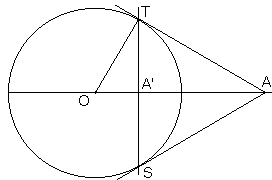# Poles and Polars

Poles and polars come in pairs. Poles are plane points; polars are straight lines in the same plane. The correspondence between points and lines that makes them into poles and polars could be defined for conic sections, including a pair of straight lines, and, more generally, for algebraic curves. Below, I define the pole/polar correspondence with respect to a circle.

So let there be a circle w with center O and radius R. Let A be an arbitrary point, except O, whose inverse image in w is A'. OA·OA' = R2. A' is collinear with O and A. Let a be the line through A' perpendicular to OA' (or OA, which is the same.) Then a is called the polar of A, while A is the pole of a, w.r.t. to w.

If A is outside w, we may draw two tangents from A to w. Then the polar a is the line through the two points of tangency. (It's this property that could be used as a definition of polar with respect to an algebraic curve.) If A lies on w, its polar a is just the tangent to w at A.

### This applet requires Sun's Java VM 2 which your browser may perceive as a popup. Which it is not. If you want to see the applet work, visit Sun's website at https://www.java.com/en/download/index.jsp, download and install Java VM and enjoy the applet.

 What if applet does not run?

The applet allows adding any number of pole/polar pairs. This can be done in two ways. You can add a pole which will appear as a small hollow circle that could be dragged. Its polar will be drawn automatically. It is also possible to add a polar - a straight (draggable) line defined by two draggable points. Its polar will then appear as a small filled circle.

Observe the main properties of poles and their polars:

1. The polar of a pole that lies inside the circle of reference lies in its entirety outsdie the circle.

2. The polar of a pole on the circle is tangent to the circle at the pole.

3. The polar of a pole that lies outside the circle of reference crosses the circle at two points. The lines joining the points to the pole are tangent to the circle.

4. If point A lies on the polar of point B, then point B lies on the polar of point A. (La Hire's Theorem.)

5. The pole of a line through two poles lies at the intersection of their polars.

6. The polar of a point which is the intersection of two polars, is the line through the corresponding poles.

7. The polars of three collinear points are concurrent.

8. The poles of three concurrent lines are collinear.

The latter properties are a direct consequence of La Hire's theorem. For example, assume points A, B, C with polars a, b, and c, respectively, lie on a straight line m. Let M be the pole of m. Since each of A, B, C lies on m, each of a, b, c passes through M. They are therefore concurrent. Conversely, let three lines a, b, c concur at point M. Then each of A, B, C lies on the polar m of M. They are therefore collinear.

Note that one needs only a straightedge to construct the polar of a point. It then follows from 3. that tangents to a circle from a point are straightedge constructible even in the absence of the center of the circle. (Compare that to Steiner's theorem.)

Let's prove 3:Let A' be the inverse image of point A lying outside the circle of inversion. A' then lies inside the circle and, by definition,

 (1) OA·OA' = R2.

Find points S and T on the circle such that ST is perpendicular to OA at A'. From (1) and the fact that they share an angle at O, triangles OAT and OTA' are similar (SAS). Since angle OA'T is right, so is angle OTA. In other words, AT is perpendicular to the radius OT and is therefore tangent to the circle at T.## Poles and Polars